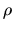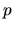Next: Polytropic Relation Up: Basic Equation of Fluid Previous: Expression for Momentum Density   Contents

# Energy Equation

The above basic equations (A.3) and (A.10) or equations (A.7) and (A.11) contain three dependent variables,, and v. The number of the variables, 3, is larger than the number of equations, 2. Therefore, an extra equation is needed to close the basic equations.

Subsections

Kohji Tomisaka 2007-07-08